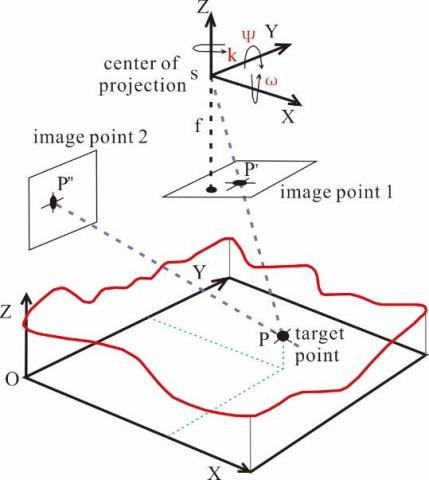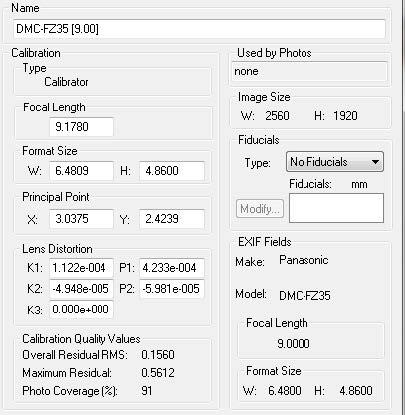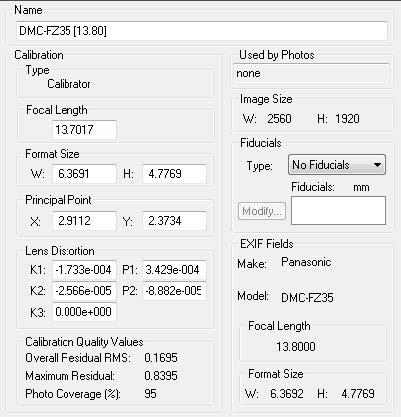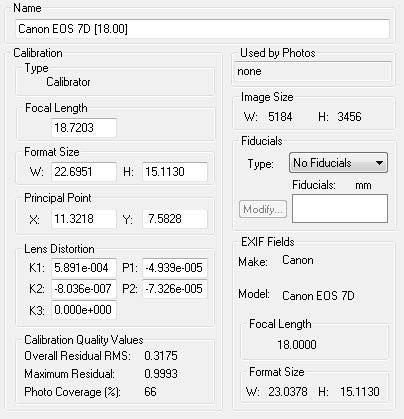# 3D Visualization of Landslide Based on Close-Range Photogrammetry

3D Visualization of Landslide Based on Close-Range Photogrammetry

Chenguang ZhangDaohan Zha Sha Zhou Hongxia Zhou Haidong Jiang

College of Geographic Sciences, Henan Key Laboratory for Synergistic Prevention of Water and Soil Environmental Pollution, Southern Henan Center for Mineral Rock and Gem-Jade Identification and Processing, Xinyang Normal University, Xinyang 464000, China

Key Laboratory of Metallogenic Prediction of Nonferrous Metals and Geological Environment Monitoring (Central South University), Ministry of Education, Changsha 410083, China

Hunan Key Lab of Land & Resource Evaluation & Utilization, Changsha 410007, China

No.3 Institute of Geo-Environment Survey of Henan, Xinyang 464000, China

School of Resources and Environmental Engineering, Guizhou University of Technology, Guiyang 550003, China

Corresponding Author Email:
Page:
479-484
|
DOI:
https://doi.org/10.18280/i2m.180507
11 May 2019
|
Accepted:
2 August 2019
|
Published:
11 November 2019
| Citation

OPEN ACCESS

Abstract:

This paper aims to accurately visualize the surface deformation of landslide. For this purpose, the authors proposed a surface modelling method based on cloud data of discrete points and triangulated irregular network (TIN). Firstly, an ordinary camera was adopted to take photos of the landslide at a high speed. Then, the close-range photogrammetry, a long-distance noncontact measurement technology, was integrated with surface modelling method, laying the basis for landslide monitoring. The hybrid method was applied to acquire the cloud data of discrete points of the landslide, reconstruct the movement pattern, and predict the future trend of movement. Lab tests show that the minimum of the largest residuals fell within 0.5612~0.9993 mm. The 3D deformation pattern reconstructed by our method in field test deviated from the actual value by less than 1mm, and from the optical measurement by less than 5mm. The results show that our method can realize accurate non-contact measurement of landslide.

Keywords:

landslide, 3D reconstruction, close-range photogrammetry, cloud data, triangulated irregular network (TIN)

1. Introduction

Photogrammetry refers to the use of camera technology to capture the image of an object, and accurately identify the shape and position of the object. The first attempt to automatize photogrammetry was made in 1950, when the Engineer Research and Development Center (ERDC) cooperated with an optical instrument company to develop an automatic photogrammetry system. Since the 1990s, photogrammetry has entered the era of full digital automation. Many digital photogrammetry systems (e.g. Virtuoso Workstation) have been applied in production and commercial services. These systems generally convert the gray values of the image into electrical signals, and then use electronic technology to realize automation.

The multi-view photogrammetry is a cutting-edge digital photogrammetry technique. Based on a group of images captured by ordinary digital cameras, the 3D structure of the real scene and the position and direction of each camera can be reconstructed under geometric constraints. The key to multi-view photogrammetry is surface modeling. The surface modelling mainly deals with non-uniform rational basis spline (NURBS) surface, triangular surface and polyhedral surface [1-4].

The triangulated irregular network (TIN), a popular surface modelling technique, is a representation of a continuous surface consisting entirely of triangular facets. The TIN model has a unique advantage: the ability to describe the surface at different levels of resolution [5-6]. Compared with the grid data model, the TIN model can describe complex surface at any resolution accurately in a short time, especially the terrains with many tectonic and fracture lines [7-9]. Therefore, this model provides a suitable tool to monitor surface deformations.

The traditional techniques for surface deformation monitoring mainly include geodetic survey and close-range photogrammetry [10-12]. In recent years, the sensor technology, GPS, and 3D laser scanning have been applied to monitor the deformation on engineering slopes, but the application faces many limitations [13-16]. Table 1 compares different minoring methods for surface deformations.

Table 1. Comparison of monitoring methods for surface deformations

 Monitoring methods Precision Operability Cost Total station, precision level High precision, 2~5mm+2ppm for total station and 0.01mm for precision level Easy to operate, with high labor intensity Low GPS, remote sensing High precision, high disturbance Easy to operate, intelligent, and efficient High Traditional close-range photogrammetry General Easy to collect field data, complex to reconstruct the 3D model of the interior Low

This paper proposes a surface modelling method based on cloud data of discrete points and the TIN for visualize the surface deformation of landslide. The TIN was integrated with the close-range photogrammetry, a long-distance noncontact measurement technology, and applied to acquire the cloud data of discrete points of the landslide, reconstruct the 3D surface and predict the future trend of movement [17-21].

2. Principle of TIN Surface Modeling

The TIN is a mature tool for digital modelling of terrains. In TIN modelling, the surface of each terrain is described by a series of interconnected triangular surfaces, which do not interest or overlap each other.

The most prominent advantage of the TIN lies in its ability to describe terrain surfaces at different levels of resolutions. The traditional grid data model cannot represent complex surfaces in a limited space within a short time. This defect is fully resolved through TIN modelling, whichever is the required resolution for the model. The TIN takes full account of the fault lines and structural lines of the target terrain, and thereby provides an accurate and reasonable description of the shape of the terrain surface. To sum up, the TIN enjoys high accuracy, fast speed and high efficiency, and the ability to deal with fault lines and other terrain features.

The TIN relies on Delaunay triangulation for terrain fitting. This triangulation approach has the best fitting results for various terrains. In the TIN model, the modelling quality hinges directly on the geometry of triangles. In general, long and narrow triangles have better interpolation effects than regular triangles, for they can better reflect the anisotropy of the terrain and the autocorrelation of space. The triangles in the TIN are unique triangles that are approximately regular, yet long and narrow. The triangles are created by linking up the nearest points.

The data of the Tin have a typical vector structure. The storage and organization structures are efficient to explicitly or implicitly express the topological relationship of scattered points on the target terrain as the relationship among nodes, triangle edges and triangle faces.

In addition, TIN modelling must follow six principles, namely, space circumcircle criterion, maximum and minimum angle criterion, minimum distance criterion, maximum angle criterion, area ratio criterion and diagonal criterion. Under these principles, the TIN can be estbalished accoridng to the original elevation points that are randomly distirbuted on the surface of the target terrain.

The TIN is useful for the description and analysis of general horizontal distributions and relationships. The network represents the topological relation between discrete points explicitly or implicitly, based on the relations between triangular nodes, edges and planes. With an efficient storage and organizational structure, the TIN obeys the following principle in triangular partitioning: the meshing quality directly hinges on the geometric shape of TIN triangles. Considering the anisotropy and spatial autocorrelation of terrains, the interpolation precision of long skinny triangles is lower than that of regular triangles. Therefore, the TIN triangles should be as equiangular as possible. Then, the most adjacent nodes connected to each other can form the only triangular. On this basis, the triangularization can be achieved at a high efficiency.

3. Calculation Principle of Space Coordinates

Based on the parameters of the digital camera and the patterns of image points, the close-range photogrammetry calculates the 3D coordinates of the target point based on optical principles and the positions of known image positions.

Let $P(X, Y, Z)$ be the coordinates of a point in space, and $P^{\prime}\left(x_{-} 1, \quad y_{-} l\right) \quad$ and $\quad P^{\prime \prime}\left(x_{-} 2, y_{-} 2\right)$ be the coordinates of its projections in two photos, respectively. As shown in Figure 1, the position $S\left(X_{s}, Y_{s}, Z_{s}\right)$  of the camera that shot the photos was taken as the projection center. The three angles between the target point and the three axes in the camera’s cordinate system are denoted as $\omega, \varphi$ and $k$, respecitvely. The focal length of the camera was denoted as f.

## 1.jpgFigure 1. The sketch map of the coordinate system

Since $P, P^{\prime}\left(P^{\prime \prime}\right)$ and S are in the same line, their relationships can be expresed as follows based on the collinearity equation:

$x_{i}=-f \cdot \frac{r_{11}\left(X-X_{S}\right)+r_{12}\left(Y-Y_{S}\right)+r_{13}\left(Z-Z_{S}\right)}{r_{31}\left(X-X_{S}\right)+r_{32}\left(Y-Y_{S}\right)+r_{33}\left(Z-Z_{S}\right)}$   (1)

$y_{i}=-f \cdot \frac{r_{21}\left(X-X_{s}\right)+r_{22}\left(Y-Y_{s}\right)+r_{23}\left(Z-Z_{s}\right)}{r_{31}\left(X-X_{s}\right)+r_{32}\left(Y-Y_{s}\right)+r_{33}\left(Z-Z_{s}\right)}$  (2)

where, i=1,2; rij can be described as:

$R\left(\omega_{i}, \varphi_{i}, k_{i}\right)=R\left(\omega_{i}\right) R\left(\varphi_{i}\right) R\left(k_{i}\right)=\left[\begin{array}{ccc}{r_{11}} & {r_{12}} & {r_{13}} \\ {r_{21}} & {r_{22}} & {r_{23}} \\ {r_{31}} & {r_{32}} & {r_{33}}\end{array}\right]$   (3)

where,

$R\left(\omega_{i}\right)=\left[\begin{array}{ccc}{1} & {0} & {0} \\ {0} & {\cos \omega_{i}} & {\sin \omega_{i}} \\ {0} & {-\sin \omega_{i}} & {\cos \omega_{i}}\end{array}\right]$

$R\left(\varphi_{i}\right)=\left[\begin{array}{ccc}{\cos \varphi_{i}} & {0} & {-\sin \varphi_{i}} \\ {0} & {1} & {0} \\ {\sin \varphi_{i}} & {0} & {\cos \varphi_{i}}\end{array}\right]$

$R\left(k_{i}\right)=\left[\begin{array}{ccc}{\cos k_{i}} & {\sin k_{i}} & {0} \\ {-\sin k_{i}} & {\cos k_{i}} & {0} \\ {0} & {0} & {1}\end{array}\right]$

The collinearity equation alone cannot determine the 3D coordinates of the point P. However, the above expression shows that P and the two projection points $S^{\prime}$ and $S^{\prime \prime}$ belong to the same plane. Then, the collinearity between them can be described as:

$F=\left|\begin{array}{lll}{b_{x}} & {b_{y}} & {b_{z}} \\ {u_{1}} & {v_{1}} & {w_{1}} \\ {u_{2}} & {v_{2}} & {w_{2}}\end{array}\right|=0$   (4)

where,

$\left[\begin{array}{l}{u_{i}} \\ {v_{i}} \\ {w_{i}}\end{array}\right]=\left[\begin{array}{ccc}{1} & {-k_{i}} & {-\varphi_{i}} \\ {k_{i}} & {1} & {-\omega_{i}} \\ {\varphi_{i}} & {\omega_{i}} & {1}\end{array}\right]\left[\begin{array}{c}{x_{i}} \\ {y_{i}} \\ {-f}\end{array}\right] i=1,2$

where, $\left[\begin{array}{l}{b_{x}} \\ {b_{y}} \\ {b_{z}}\end{array}\right]$ is the vector between en $S^{\prime}$ and $S^{\prime \prime}$.

4. LAB Test

4.1 Camera calibration

Before the close-range photogrammetric monitoring, it is necessary to calibrate the camera on the software, and store the calibrated parameters as data files. First, the photos shot by the camera, which contain camera parameters and dynamic parameters of the shooting process, were inputted to the software. Then, the software would identify the parameters in the photos, find the corresponding camera parameters in the data file, and perform back calculation with both of them. If the camera was used without calibration, the software would receive errors during the identification process.

The camera was calibrated with a 630cm-long square calibration board. There are 100 equidistant calibration points on the board, with the interval of 70mm. Each calibration point is 9mm in diameter. Four control points were selected on the board, with the inner diameter of 9mm and outer diameter of 38mm. In this paper, the focal length of the camera is adjusted to that of Panasonic DMC-FZ35 or Canon EOS 7D before shooting.

During the calibration (Figure 2), the digital camera was erected to shoot the calibration board from four directions at 90° directions. All the calibration points must appear in each photo, covering no less than 70% of the whole photo. Then, the camera was rotated counterclockwise by 90° for another round of shooting. Finally, the camera was rotated clockwise by 90° for the last round of shooting. In total, 12 photos were taken at each focal length. The photos were analyzed to evaluate the calibration results (Figures 3-5).

## 2.jpgFigure 2. The calibration processes

The calibration results in Figures 3-5 show that the maximum residual minimized at 0.56 (Panasonic DMC-FZ35 [9.00mm]). According to the user manual of the PMS software, the maximum residual should be less than 1. Therefore, smaller the residual, the better the calibration precision. With a zoom length of 18~135 mm, the focal length of Canon EOS 7D is easy to control. However, its response time is too short for photo collection. To minimize the residual, this paper selects the calibration results of Canon EOS 7D at the focal length of 18mm for lab test and field test.

## 3.jpgFigure 3. Calibration results for Panasonic DMC-FZ35 [9.00]

## 4.jpgFigure 4. Calibration results for Panasonic DMC-FZ35 [13.80]

## 5.jpgFigure 5. Calibration results for Canon EOS 7D [18.00]

4.2 Model testing

After calibration, a digital camera (DC) and a digital single-lens reflex (DSLR) camera were tested on a calibration board (Figure 6). On the board, there are 9 calibration points of the same size. The cap of each point is black except for a white dot in the center. The first eight points were arranged at the vertical interval of 25 cm and the lateral interval of 15 cm. The last point was placed at the rightmost with the vertical interval of 12.5 cm and the lateral interval of 15 cm. All these points have the same vertical coordinate. Each calibrated camera (with the focal length of 18 mm) shot eight photos of the calibration board at different focal lengths from varied directions. The photos were then analyzed on the PMS software to reconstruct the data of the nine calibration points.

## 6.jpgFigure 6. Layout of the calibration points

Figure 7 shows the calibration results of Cannon EOS7D (focal length: 18 mm). The three break lines represent the deviation of the reconstructed position from the actual position of each calibration point in the x-, y-, and z- directions. It can be seen that the maximum deviation was smaller than 1mm. Thus, the calibrated camera achieved the monitoring precision for engineering slope.

## 7.jpgFigure 7. The calibration results of Cannon EOS7D

5. Field Test

After the lab test, the authors conducted a field test on a slope in Changsha, southern China’s Hunan province. First, the point cloud data of the slope were collected through close-range photogrammetry (Figure 8). Then, the surface of the landslide on the slope was modelled by the TIN (Figure 9). The PMS data were compared with the data measured by a third party (Figure 10).

## 8.jpgFigure 8. The point cloud data of the slope

## 9.jpgFigure 9. The TIN modelling of landslide surface

As shown in Figure 10, the maximum error between the PMS data and the third-party data was within 5%, which satisfies the precision requirement for engineering measurement. The results demonstrate the speed, efficiency and real-time performance of the TIN surface modelling.

## 10.jpgFigure 10. Comparison between data from different sources

6. Conclusions

This paper combines ordinary photographic method with close-range photogrammetry and TIN surface modelling into a novel approach to measure the surface deformation of landslide. This approach can collect the cloud data points, reconstruct the movement pattern, and predict the movement trend of the landslide. Through theoretical analysis, lab test and field test, the following conclusions were put forward:

(1) Based on the principle of TIN surface modelling, the authors established a non-contact close-range photogrammetry and back-solving model for landslide.

(2) The calibration test shows that, after TIN surface modeling, the maximum residual was minimized at 0.56 mm for Panasonic DMC-FZ35 [9.00 mm]. The error was smaller than the 1 mm threshold, fulfilling the precision requirement for engineering measurement.

(3) In field test, the point cloud data of the slope were collected through close-range photogrammetry, and the surface of the landslide on the slope was modelled by the TIN. The deviation of the PMS data from the actual data was controlled within 5 mm. The results verify the feasibility of TIN surface modeling in deformation monitoring of landslide.

(4) The lab test and field test show that, in addition to predict the trend of landslide, our method has the potential to analyze the cause and failure mechanism of landslide.

Acknowledgment

This research is supported by Key Scientific and Technological Research Project of Henan Province (Grant No.: 192102310268), Open Research Fund Program of Key Laboratory of Metallogenic Prediction of Nonferrous Metals and Geological Environment Monitoring (Central South University), Ministry of Education (Grant No.: 2019YSJS08), Nanhu Scholars Program for Young Scholars of Xinyang Normal University, Special Projects for Promoting Big Data Development of Guizhou Institute of Technology, and Geological Resources and Geological Engineering, Guizhou Provincial Key Disciplines (Grant No.: ZDXK 001).

References

 Snavely, N., Seitz, S.M., Szeliski, R. (2008). Modeling the world from internet photo collections. International Journal of Computer Vision, 2(80): 189-210. http://doi.org/10.1007/s11263-007-0107-3

 Kahl, F., Agarwal, S., Chandraker, M.K., Kriegman, D., Belongie, S. (2008). Practical global optimization for multiview geometry. International Journal of Computer Vision, (3): 592-605. http://doi.org/10.1007/s11263-007-0117-1

 Kahl, F., Henrion, D. (2007). Globally optimal estimates for geometric reconstruction problems. International Journal of Computer Vision, 1(74): 3-15. http://doi.org/10.1007/s11263-007-0117-1

 Ke, Y.L., Xiao, Y., Li, J.X. (2001). Research on reversing engineering by CAD modeling techniques. Journal of Computer-Aided Design and Computer Graphics, 13(6): 570-576. http://doi.org/10.3321/j.issn:1004-132X.2001.z1.011

 Bradley, C.H., Vickers, G.W. (1993). Free-form surface reconstruction for machine vision rapid prototyping. Optical Engineering, 32(9): 2191-2200. http://doi.org/10.1117/12.145064

 Sui, L.S., Jiang, Z.D. (2003). Raster-type free-form surface modeling technology research of point cloud data. Journal of Computer-Aided Design and Computer Graphics, 10(15): 1293-1297. http://doi.org/10.3321/j.issn:1003-9775.2003.10.019

 Zheng, J.D. (2013). Key technology research and theory of three-dimensional photogrammetry based on digital camera. Nanjing Aeronautics and Astronautics University, 1-6.

 Huang, S., Yin, H., Jiang, Z. (2011). Deformation monitoring data processing. Wuhan University Press, 1-8. http://doi.org/10.1007/bf02826929

 Scaioni, M., Feng, T.T., Lu, P., Qiao, G., Tong, X.H., Li, R., Barazzetti, L., Previtali, M., Roncella, R. (2015). Close-Range photogrammetric techniques for deformation measurement: applications to landslides. Modern Technologies for Landslide Monitoring and Prediction, 13-41. http://doi.org/10.1007/978-3-662-45931-7_2

 Matori, A.N., Cahyono, B.K., Mokhtar, M.R.M., Yusof, K.B.W. (2013). Close-range photogrammetric data for landslide monitoring on slope area. Humanities, Science & Engineering. http://doi.org/10.1109/CHUSER.2012.6504346

 Mokhtar, M.R.M., Matori, A.N., Khamaruzaman, H.W.Y.H., Chandio, I.A., Viet, D.T., Lawal, D.U. (2012). A study of unmanned aerial vehicle photogrammetry for environment mapping: preliminary observation. Advanced Materials Research, 626: 440-444. http://doi.org/10.4028/www.scientific.net/AMR.626.440

 Travelletti, J., Delacourt, D., Allemand, P., Malet, J.P., Schmittbuhl, J., Toussaint, R., Bastard, M. (2012). Correlation of multi-temporal ground-based optical images for landslide monitoring: Application, potential and limitations. Journal of Volcanology & Geothermal Research, 70(3-4): 39-55. http://doi.org/10.1016/j.isprsjprs.2012.03.007

 Deparis, J., Fricout, B., Jongmans, D., Villemin, T., Effendiantz, L., Mathy, A. (2008). Combined use of geophysical methods and remote techniques for characterizing the fracture network of a potentially unstable cliff site (the ‘Roche du Midi’, Vercors massif, France). Journal of Geophysics & Engineering, 5(2): 147-157. http://doi.org/10.1088/1742-2132/5/2/002

 Fernandez, T., Jimenez, J., Fernandez, P., El Hamdouni, R., Cardenal, F.J., Delgado, J., Irigaray, C., Chacón, J. (2008). Automatic Detection of Landslide Features With Remote Sensing Techniques in the Betic Cordilleras (Granada, Southern Spain). ISPRS Congress.

 Chidburee, P., Mills, J.P., Miller, P.E., Fieber, K.D. (2016). Towards a low-cost real-time photogrammetric landslide monitoring system utilising mobile and cloud computing technology. ISPRS - International Archives of the Photogrammetry, Remote Sensing and Spatial Information Sciences, XLI-B5: 791-797. http://doi.org/10.5194/isprsarchives-XLI-B5-791-2016

 Brideau, M.A., Sturzenegger, M., Stead, D., Jaboyedoff, M., Lawrence, M., Roberts, N.J., Ward, B.C., Millard, T.H., Clague, J. (2012). Stability analysis of the 2007 Chehalis lake landslide based on long-range terrestrial photogrammetry and airborne LiDAR data. Landslides 9(1): 75-91.

 Torné, M., Guinau, M., Tapia, M., Perez, C., Jesús, R.M., Echeverria, A., Roig, P., Suriñach, E. (1995). Reconstructing landslide dynamics and characteristics using remote sensing data (photogrammetry, lidar and seismic data): Comparison between different techniques and complementary data analysis. Journal of Shoulder & Elbow Surgery, 4(95): S83. http://doi.org/10.1016/S1058-2746(95)80288-6

 Barbarella, M., Fiani, M., Lugli, A. (2014). Application of lidar-derived dem for detection of mass movements on a landslide. ISPRS - International Archives of the Photogrammetry, Remote Sensing and Spatial Information Sciences volume XL-5/w3: 159-165. http://doi.org/10.5194/isprsannals-II-5-65-2014

 Nichol, J., Wong, M.S., Shaker, A. (2009). Application of high-resolution satellite images to detailed landslide hazard assessment. Urban Remote Sensing Event 2009. http://doi.org/10.1109/URS.2009.5137737

 Peng, D.L., Xu, Q., Dong, X.J., Ju, Y.Z., Qi, X., Tao, Y.Q. (2017). Application of Unmanned Aerial Vehicles Low-altitude Photogrammetry in Investigation and Evaluation of Loess Landslide. Advances in Earth Science (2017). http://doi..org/10.11867/j.issn.1001-8166.2017.03.0319

 Gabrieli, F., Corain, L., Vettore, L. (2016). A low-cost landslide displacement activity assessment from time-lapse photogrammetry and rainfall data: Application to the Tessina landslide site. Geomorphology, 269: 56-74. http://doi..org/10.1016/j.geomorph.2016.06.030